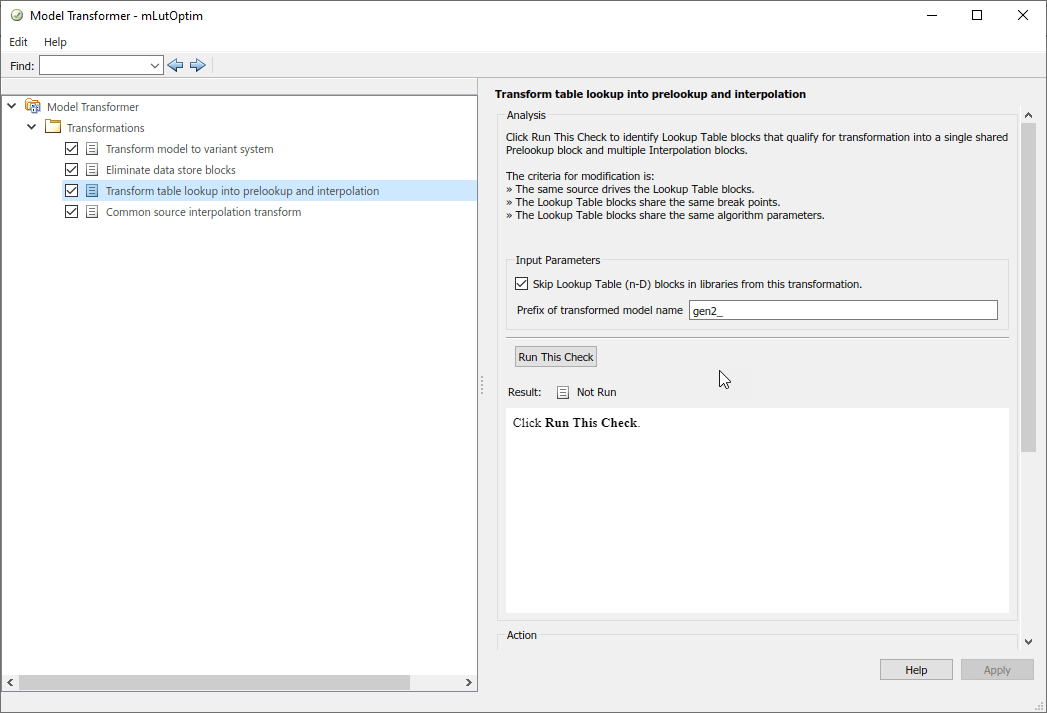## Improve Efficiency of Simulation by Optimizing Prelookup Operation of Lookup Table Blocks

Improve the efficiency of your model simulation by using the Model Transformer tool to identify n-D Lookup Table blocks that qualify for transformation and replacing them with Interpolation blocks and shared Prelookup blocks. Eliminating the redundant Prelookup blocks improves the simulation speed for linear interpolations. The Model Transformer creates a model with these replacements blocks. This new model has the same functionality as the original model.

The Model Transformer can replace Lookup Table blocks that meet the following conditions:

• The same source drives the Lookup Table blocks.

• The Lookup Table blocks share the same breakpoint specification, values, and data types.

• The breakpoint input port of the Lookup Tables is the connected to the same input source.

• The Lookup Table blocks share the same algorithm parameters in the block parameters dialog box.

• The Lookup Table blocks share the same data type for fractions parameters in the block parameters dialog box.

### Improve Efficiency of Simulation by Optimizing Prelookup Operation of Lookup Table Blocks Using Model Transformer

This example shows how to use Model Transformer to identify Lookup Table blocks that qualify for transformation and replacing them with Interpolation blocks and shared Prelookup blocks.The model `ex_lut_optimize` contains three Lookup Table blocks: LUT1, LUT2 and LUT3. The blocks are driven from the same input sources In1 and In2.

Merge Prelookup Operation

Identify n-D Lookup Table blocks that qualify for transformation and replace them with a single shared Prelookup block and multiple Interpolation blocks.

1. Open the model `ex_lut_optimize`.

2. Save the model to your working folder.

3. On the Apps tab, click Model Transformer.

4. In the Transformations folder, select the Transform Table Lookup Blocks to Prelookup and Interpolation Using Prelookup Blocks check.

5. Select the Skip Lookup Table (n-D) blocks in the libraries from this transformation option to avoid replacing Lookup Table blocks that are linked to a library.

6. In the Prefix of refactored model field, specify a prefix for the new refactored model.

7. Click the Run This Check button. The top Result table contains hyperlinks to the Lookup Table blocks and the corresponding input port indices.

8. Clear the Candidate Groups that you do not want to transform.

9. Click the Refactor Model button. The Result table contains a hyperlink to the new model. The table also contains hyperlinks to the shared Prelookup block and corresponding Interpolation blocks. Those blocks replaced the original Lookup Table blocks. The tool creates an `m2m_ex_lut_optimize` folder. This folder contains the new `gen_ex_lut_optimize.slx` model.The Lookup Table blocks LUT1, LUT2, and LUT3 of `gen_ex_lut_optimize.slx` have two shared Prelookup table blocks, `LUT1_Prelookup_1` and `LUT1_Prelookup_2`, one for each data source. There are also three Interpolation blocks `LUT1_InterpND`, `LUT2_InterpND`, and `LUT3_InterpND` that replace the Lookup Table blocks.### Conditions and Limitations

The Model Transformer cannot replace Lookup Table blocks if:

• A Rate Transition block drives the Lookup Table blocks.

• The Lookup Table blocks are commented-out regions and inactive variants.

• The Lookup Table blocks are masked.

• The Output block's data type is set to `Inherit:Same as first input`.

• The Lookup Table block Interpolation method and Extrapolation method on the Algorithm pane of the block parameters dialog box is set to `Cubic spline`.

• The Lookup Table block Input settings on the Algorithm pane of the block parameters dialog box has Use one input port for all input data selected.

The Lookup Table block Code generation on the Algorithm pane of the block parameters dialog box has Support tunable table size in code generation selected.

The Model Transformer tool does not replace Lookup Table blocks across the boundaries of Atomic subsystems, Referenced Models, and library-linked blocks.